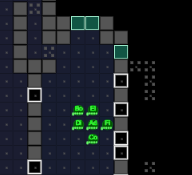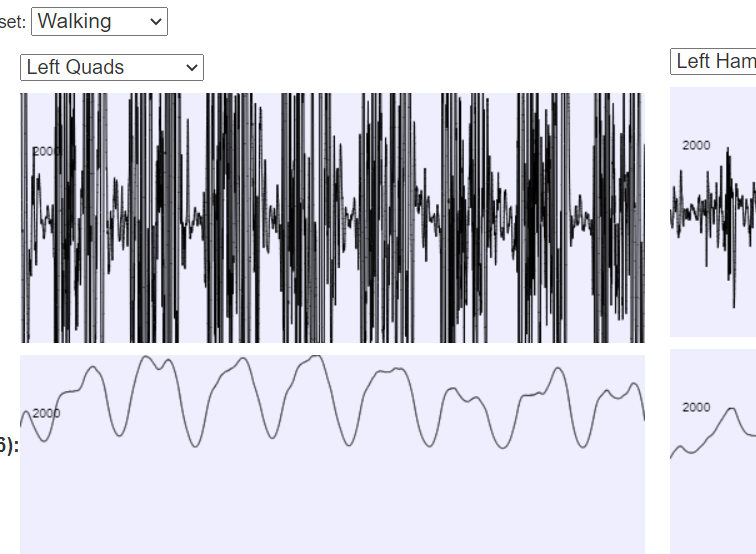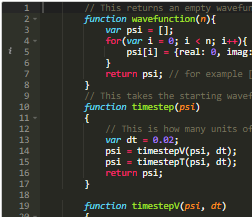﻿ How SpaceX land first stage boosters Part 2
﻿

# How SpaceX land first stage boosters Part 2 --- neural networks and technology discussion.

In the previous article, here, I went over how a conical optimiser can be used to plan optimal trajectories.

The main problem with this so far are that it's slow - it took around a second to plan a trajectory, and this needs to be redone frequently: Ideally several times a second.

To get round this problem, one solutions is to do the following:
• Do hundreds of simulations using the conical solver to control the rocket.
• Record the state of the rocket and what the conical solver said at each timestep.
• Train a neural network to predict what the conical solver is going to mandate.
This isn't something that I think SpaceX has done, but it's very simple, and works for me to some extent:

The reasons that it's not great for SpaceX are probably:
• There are no guarantees that it's going to actually work. It might crash.
• It's not going to be optimal. It might run out of fuel ... and then crash.
For me, though, there's one big advantage of using a neural network here: The neural network can be easily ported to javascript --- the conical solver cannot.

Here's an example:

AI status:
Off.
Use keys W,A,D to control the rocket manually. 'c' toggles AI

Note that this isn't optimal. You can kind-of see that it sort-of works, but it's not perfect at all. It sometimes bounces on the ground on the way to a successful landing.

## Kotlin

To me, Kotlin is a great language for this kind of research: It's concise and fast. It might not be as good for the fastest applications, or for applications that need tight memory controls or need to avoid garbage collecting at bad times, but if you're writing a quick script to do something like simulate a rocket launch, it's excellent.
For an example, here's the entire 3-vector library that I use in my simulation. There are very few languages that could express these functions as clearly and concisely. Even if you would normally use a library for 3-vectors, the point still stands that Kotlin is extremely clear and concise:

class V3(var x:Double=0.0, var y:Double=0.0, var z:Double=0.0){
operator fun minus(a : V3) = V3(x - a.x, y - a.y,z - a.z)
operator fun plus(a : V3) = V3(x + a.x, y + a.y,z + a.z)
operator fun times(dt: Double) = V3(x*dt,y*dt,z*dt)
operator fun rem(a:V3) = Math.sqrt((x-a.x)*(x-a.x) + (y-a.y)*(y-a.y) + (z-a.z)*(z-a.z))
operator fun times(a:V3) = V3(y * a.z - z * a.y, z * a.x - x * a.z, x * a.y - y * a.x)

fun length2() = x*x + y*y + z*z
fun length() = Math.sqrt(x*x+y*y+z*z)
infix fun dot(d: V3) = x*d.x + y * d.y + z*d.z
override fun toString() = "($x,$y,$z)" fun copy() = V3(x,y,z) fun addAndScaleIP(d: V3, dt: Double) { x+=d.x * dt y+=d.y * dt z+=d.z * dt } fun addIP(d: V3) { x+=d.x y+=d.y z+=d.z } fun scale(dt: Double) = V3(x*dt,y*dt,z*dt) fun isEqual(v3: V3) = x==v3.x&&y==v3.y&&z==v3.z fun normalise() = scale(1.0 / this.length()) fun isFinite() = x.isFinite() && y.isFinite() && z.isFinite() fun toPov() = "<$x,$y,$z >"
fun toDoubleArray() = doubleArrayOf(x,y,z)

}

fun main(args:Array< String >){
val a = V3(1.2,2.5,-0.2)
val b = V3(1.6,-2.5,0.2)

println("a=$a") println("b=$b")
val c = a + b * 2.0    // scaling
val d = a dot c        // infix operators
val e = a * b + V3(d,0.0,0.0)          // cross product

println("a + b = $c") println("a + b =${a+b}")
println("e = $e") }  ## Tensorflow and neural networks I trained the neural networks here using python/tensorflow: import tensorflow as tf import numpy as np import json import matplotlib.pyplot as plt files = [ "C:/data/tfSimple6dofH.json", "C:/data/tfSimple6dofI.json", "C:/data/tfSimple6dofJ.json", "C:/data/tfSimple6dofK.json", "C:/data/tfSimple6dofL.json", "C:/data/tfSimple6dofM.json", "C:/data/tfSimple6dofN.json", "C:/data/tfSimple6dofO.json", "C:/data/tfSimple6dofP.json" ] datasets = [json.load(open(_)) for _ in files] dataset = [] for ds in datasets: for d2 in ds: dataset.append(d2) print("length of dataset = " + str(len(dataset)) + " rows") cutoff = (len(dataset)*3)//4 train = dataset[:cutoff] test = dataset[cutoff:] x_train = np.array([_['input'] for _ in train]) y_train = np.array([_['output'] for _ in train])*0.01+0.5 x_test = np.array([_['input'] for _ in test]) y_test= np.array([_['output'] for _ in test])*0.01+0.5 model = tf.keras.models.Sequential([ tf.keras.layers.Dense(20, activation=tf.nn.sigmoid), tf.keras.layers.Dense(20, activation=tf.nn.sigmoid), tf.keras.layers.Dense(len(train['output']), activation=tf.nn.sigmoid) ]) model.compile(optimizer='adam', loss='mse'#'sparse_categorical_crossentropy', #metrics=['accuracy'] ) scoreIn = [] scoreOut = [] for i in range(1000): model.fit(x_train, y_train, epochs=5, verbose=10, steps_per_epoch=5) a = model.evaluate(x_train, y_train) b = model.evaluate(x_test, y_test) print("iteration " + str(i) + " score = " + str(int(a*1e5)) + " vs " + str(int(b*1e5))+"\n") scoreIn.append(a) scoreOut.append(b) w=model.get_weights() j = [] for i in range(len(w)//2): j.append({ 'weights': w[i*2+0].tolist(), 'bias': w[i*2+1].tolist() }) json.dump({'network':j}, open("C:/tmp/weights6dofB.json",'w')) plt.plot(scoreOut[10:]);plt.plot(scoreIn[10:])  This isn't really complicated at all. It may have been better, in an ideal world, to use Kotlin throughout or python throughout. ## The actual optimizer I used ecos as the conical optimizer. It's written in C, and I got it to compile and run under Windows using Cygwin. I wrote a quick program in C to load a problem from a text file, solve it, and put the solution in a text file. It would have been better to use pipes or something, but there's a time and a place for just getting results quickly. I have to say that the documentation, at least in the current version, for ECOS, is hard to use. The C interface is only described in one paragraph that doesn't tie the variable names to the equivalent equations properly, and which overrun the edge of the PDF page. The way to learn to use it is to start off with the simplest possible problem, and then keep altering it until you get a sensible result, and then keep adding to it until you end up with a list of what's what. ## Kotlin problem generation There was a comment asking for more detail about how to construct a conical solver problem from the trajectory constraints. Here's the code. Note that the variables have the same names as the C code, but the output format isn't a format that ECOS accepts - you have to write a layer in C or something that reads this and calls the ecos methods. However, I think the code below is fairly straightforward, and so it should be helpful for anyone trying to use ECOS for this purpose. class Problem{ var nVars : Int = 0 val G = mutableListOf< DoubleArray>() val q = mutableListOf< Int>() val c = mutableListOf< Double>() val h = mutableListOf< Double>() var pEquals = 0 var mConeVars = 0 var lOrthants = 0 var sCones = 0 fun save(fn : String){ val ret = mutableListOf< String>() ret.add("(n$nVars)")
ret.add("(p $pEquals)") ret.add("(m$mConeVars)")
ret.add("(l $lOrthants)") ret.add("(s$sCones)")
val gMatrix = newMatrix(G)
val gNonZero = gMatrix.nonZeros()
ret.add("(g $gNonZero)") ret.add("(end)") ret.add("") for(i in 0 until q.size){ ret.add("(q$i,${q[i]})") } for(i in 0 until c.size){ ret.add("(c$i,${c[i]})") } for(i in 0 until h.size){ ret.add("(h$i,${h[i]})") } for(i in 0 until G.size){ for(j in 0 until G[i].size){ val g2 = G[i][j] if (g2!=0.0){ ret.add("(g$i,$j,$g2)")
}
}
}
ret.save(fn)
}
this.nVars++
return this.nVars-1
}
if (sCones>0) throw Exception("Add orthants before cones")
lOrthants++
mConeVars++
}
sCones++
mConeVars+=g.size
}

fun constrainTwice(i: Int, x: Double, tolerance:Double=0.0) {
val d = DoubleArray(nVars)
d[i] = 1.0
val d2 = DoubleArray(nVars)
d2[i] = -1.0
}

}

class trajectoryInput(
var pos:V3,
var vel: V3,
var g:Double,
var dt:Double,
var nSteps:Int,
var verticalTime:Double,
var maxThrust: Double)

class ConeOptions(var justDown : Boolean, var endingFall : Boolean)

fun coneTrajectory(inp:trajectoryInput, coneOptions: ConeOptions) : Problem{
/*

Variables:

velocities in each step
abs thrust used in each step

change in velocity must be less than abs thrust used.
minimise total abs thrust
abs thrust must be less than threshold.

*/
var prob = Problem()
var vx = mutableListOf< Int>()
var vy = mutableListOf< Int>()
var vz = mutableListOf< Int>()
var thrust = mutableListOf< Int>()
for (i in 0 until inp.nSteps) {
if (i < inp.nSteps - 1) {
}
}
val dai = { DoubleArray(prob.nVars) }

prob.constrainTwice(vx, inp.vel.x)
prob.constrainTwice(vy, inp.vel.y)
prob.constrainTwice(vz, inp.vel.z)

var driftTolerance = 0.3
prob.constrainTwice(vx[inp.nSteps - 1], 0.0, driftTolerance)
prob.constrainTwice(vy[inp.nSteps - 1], 0.0, driftTolerance)
prob.constrainTwice(vz[inp.nSteps - 1], 0.0, driftTolerance)

if (coneOptions.endingFall) {
// for the last few steps, I only want vertical movement.
val verticalSteps = Math.min(inp.nSteps, (inp.verticalTime / inp.dt).toInt())
val acceleration = inp.maxThrust + inp.g // g negative, so this'll be about 20m/s normally.

for (i in inp.nSteps - verticalSteps until inp.nSteps - 1) {
prob.constrainTwice(vx[i], 0.0, driftTolerance)
prob.constrainTwice(vz[i], 0.0, driftTolerance)

if (i == inp.nSteps - verticalSteps) {
val timeToEnd = (inp.nSteps - i) * inp.dt
val height = -0.5 + 0.5 * timeToEnd * timeToEnd * acceleration
val dMinH = dai()

dMinH[vy[i]] = -0.5
for (j in i until inp.nSteps - 1) {
dMinH[vy[j]] = -1.0
}
// dMinH is now the height at this timestep
prob.addOrthant(dMinH * -1.0, height * -1.0)
}
}
}
// The sum of the velocities must be equal to the negative of initial position, so that it ends up at 0.
val dx = dai()
val dy = dai()
val dz = dai()
dx[vx] = inp.dt * 0.5
dy[vy] = inp.dt * 0.5
dz[vz] = inp.dt * 0.5
for (i in 1 until inp.nSteps - 1) {
dx[vx[i]] = inp.dt
dy[vy[i]] = inp.dt
dz[vz[i]] = inp.dt
}
dx[vx[inp.nSteps - 1]] = inp.dt * 0.5
dy[vy[inp.nSteps - 1]] = inp.dt * 0.5
dz[vz[inp.nSteps - 1]] = inp.dt * 0.5

var posTolerance = 2.0

for (i in 0 until inp.nSteps - 1) {
val d = dai()
d[thrust[i]] = 1.0
prob.addOrthant(d*-1.0, 0.0) // this constrains it to be positive, shouldn't be necessary.

if (coneOptions.justDown) {
// The thrust has to be upward: no boosting downwards.
val d2 = dai()
d2[vy[i]] = 1.0
d2[vy[i + 1]] = -1.0
prob.addOrthant(d2, -inp.g * inp.dt * 1)

}
}
for (i in 0 until inp.nSteps - 1) {
val dx = dai()
dx[vx[i]] = 1.0
dx[vx[i + 1]] = -1.0
val dy = dai()
dy[vy[i]] = 1.0
dy[vy[i + 1]] = -1.0
val dz = dai()
dz[vz[i]] = 1.0
dz[vz[i + 1]] = -1.0
}
return prob
}

class TrajectoryOutput(val pos : List< V3>, val vel : List< V3>, val thrust : List< V3>)

fun getTrajUnknownN(inp:trajectoryInput, coneOptions: ConeOptions) : TrajectoryOutput{
var min = 2
var max = 250
var mid = 75
for(i in 0 until 10){
inp.nSteps = mid
//println("trying \${inp.nSteps} steps" )
val ret = getTraj(inp, coneOptions)
if (ret == null){
min = mid
mid = (max+min)/2
if (mid == min) mid++
}
else{
max = mid
mid = (max + min)/2
if (mid==min || mid==max) return ret
}

}
return getTraj(inp, coneOptions)!!
}



## PD controller

There was a part of the simulation that I glossed over: the PD controller. This is a controller that knows how fast the rocket is rotating, and the direction that it is meant to be facing (and thrusting), and controls the thrusters to orient the spacecraft accordingly. I have no idea whether this is similar to what SpaceX uses, and I strongly suspect that they use something a lot more sophisticated, perhaps putting part of the angle control into the main conical problem or something. But anyway, here's this.

The output doublearray is the control vector to be sent to the thrusters. The 0'th element is the main engine on the bottom of the craft, and the remaining elements are the 4 thrusters at the top of the rocket.

class ConicControlOutput(val thrustDir : V3, val pointDir:V3, val expectedPosition : List< V3>){
fun getControl(s : Dynamics.State, d : Dynamics) : DoubleArray{
var pd = pointDir.copy().normalise()
var minCosTheta = 0.7
if (pd.y < minCosTheta){
pd.y = 0.0
pd = pd.normalise() * Math.sqrt(1 - minCosTheta*minCosTheta)
pd.y=minCosTheta

}
var target = pd *-1.0

var targetW = (s.rot.fo * target) + s.w*0.3

val ret = DoubleArray(d.thrusters.size)

for(i in 0 until d.thrusters.size){
val thruster = d.thrusters[i]
val dw = s.rot.objToAbs(thruster.dir * thruster.pos) dot targetW
var result = 0.0
if (dw > 0) result = Math.min(dw*0.03,1.0)
ret[i] = result
}

var mainThrust = thrustDir dot (s.rot.objToAbs(d.thrusters.dir))
mainThrust *= 1.0 / (d.thrusters.force / s.getMass())
if (mainThrust < 0) mainThrust=0.0
if (mainThrust > 1) mainThrust = 1.0
ret = mainThrust
return ret
}
}



## Summary

Those are a few extra details that probably won't be interesting unless you're trying to do something like this yourself.

The original article is here

## Asciiship

My latest (early 2018) thing is just a "normal" game: no real physics. It's just a game.## BodyWorks: EMG Analysis

A page with a javascript application where you can interact with EMG data using various filters.## Quantum Mechanics for programmers

A quick demo showing how to make simulations of simple quantum mechanics systems in javascript.